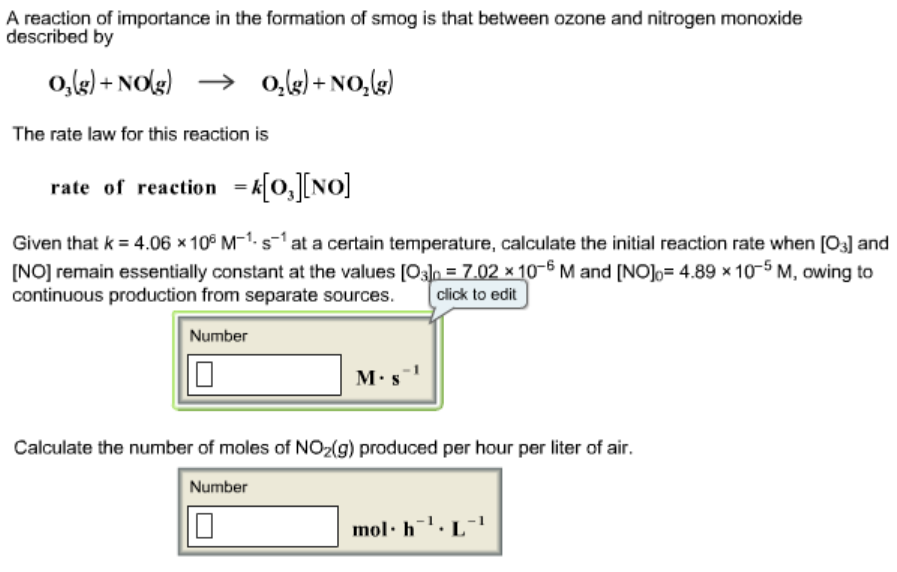# Problem: A reaction of importance in the formation of smog is that between ozone and nitrogen monoxide described byO3(g) + NO(g) --&gt; O2(g) + NO2(g)The rate law for this reaction israte of reaction = k[O3] [NO]Given that k = 4.06 x 106 M-1 s-1 at a certain temperature, calculate the initial reaction rate when [O3] and [NO] remain essentially constant at the values [O3] = 7.02 x10-6 M and [NO] = 4.89x10-5 M, owing to continuous production from separate sources.Calculate the number of moles of NO2(g) produced per hour per liter of air.

###### FREE Expert Solution
91% (273 ratings)###### Problem Details

A reaction of importance in the formation of smog is that between ozone and nitrogen monoxide described by
O3(g) + NO(g) --> O2(g) + NO2(g)

The rate law for this reaction is
rate of reaction = k[O3] [NO]

Given that k = 4.06 x 106 M-1 s-1 at a certain temperature, calculate the initial reaction rate when [O3] and [NO] remain essentially constant at the values [O3] = 7.02 x10-6 M and [NO] = 4.89x10-5 M, owing to continuous production from separate sources.

Calculate the number of moles of NO2(g) produced per hour per liter of air.